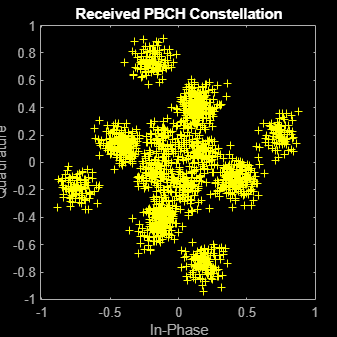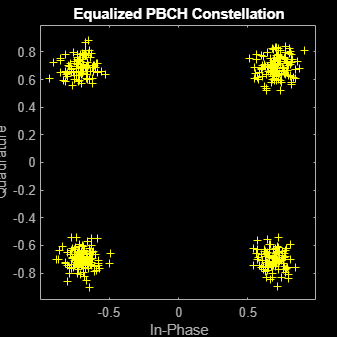nrEqualizeMMSE

Minimum mean-squared error (MMSE) equalization

Description

example

[eqSym,csi] = nrEqualizeMMSE(rxSym,hest,nVar) applies MMSE equalization to the extracted resource elements of a physical channel rxSym and returns the equalized symbols in eqSym. The equalization process uses the estimated channel information hest and the estimate of the received noise variance nVar. The function also returns the soft channel state information csi.

Examples

collapse all

Perform MMSE equalization on extracted resource elements of the physical broadcast channel (PBCH).

Create symbols and indices for a PBCH transmission.

ncellid = 146;
v = 0;
E = 864;
cw = randi([0 1],E,1);
pbchTxSym = nrPBCH(cw,ncellid,v);
pbchInd = nrPBCHIndices(ncellid);

Generate an empty resource array for one transmitting antenna. Populate the array with the PBCH symbols by using the generated PBCH indices.

carrier = nrCarrierConfig('NSizeGrid',20);
P = 1;
txGrid = nrResourceGrid(carrier,P);
txGrid(pbchInd) = pbchTxSym;

Perform OFDM modulation.

txWaveform = nrOFDMModulate(carrier,txGrid);

Create channel matrix and apply channel to transmitted waveform.

R = 4;
H = dftmtx(max([P R]));
H = H(1:P,1:R);
H = H / norm(H);
rxWaveform = txWaveform * H;

Create channel estimate.

hEstGrid = repmat(permute(H.',[3 4 1 2]),[240 4]);
nEst = 0.1;

Perform OFDM demodulation.

rxGrid = nrOFDMDemodulate(carrier,rxWaveform);

To prepare for PBCH decoding, use nrExtractResources to extract symbols from received and channel estimate grids. Plot the received PBCH constellation.

[pbchRxSym,pbchHestSym] = nrExtractResources(pbchInd,rxGrid,hEstGrid);
figure;
plot(pbchRxSym,'o:');Decode the PBCH with the extracted resource elements. Plot the equalized PBCH constellation.

[pbchEqSym,csi] = nrEqualizeMMSE(pbchRxSym,pbchHestSym,nEst);
pbchBits = nrPBCHDecode(pbchEqSym,ncellid,v);
figure;
plot(pbchEqSym,'o:');
title('Equalized PBCH Constellation');Input Arguments

collapse all

Extracted resource elements of a physical channel, specified as an NRE-by-R numeric matrix. NRE is the number of resource elements extracted from each K-by-L plane of the received grid. K is the number of subcarriers and L is the number of OFDM symbols. R is the number of receive antennas.

Data Types: double
Complex Number Support: Yes

Estimated channel information, specified as an NRE-by-R-by-P numeric array. NRE is the number of resource elements extracted from each K-by-L plane of the received grid. K is the number of subcarriers and L is the number of OFDM symbols. R is the number of receive antennas. P is the number of transmission planes.

Data Types: double
Complex Number Support: Yes

Estimated noise variance, specified as a real nonnegative scalar.

Data Types: double

Output Arguments

collapse all

Equalized symbols, returned as an NRE-by-P numeric matrix. NRE is the number of resource elements extracted from each K-by-L plane of the received grid. K is the number of subcarriers and L is the number of OFDM symbols. P is the number of transmission planes.

Data Types: double
Complex Number Support: Yes

Soft channel state information, returned as an NRE-by-P numeric matrix. NRE is the number of resource elements extracted from each K-by-L plane of the received grid. K is the number of subcarriers and L is the number of OFDM symbols. P is the number of transmission planes.

Data Types: double
Complex Number Support: Yes